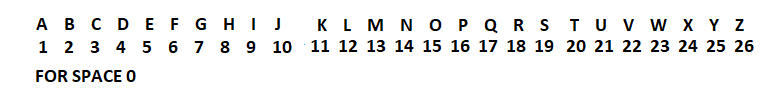# Python | Encoding Decoding using Matrix

Encoding process :

1. Take a String convert to corresponding number shown below2. convert to 2D matrix(array). Now we have 2×2 matrix!
3. When we multiply this matrix with encoding matrix we get encoded 2×2 matrix.
4. now convert to vector(1D array) and Display to user

Decoding process

• Take Encoded number convert into 2D matrix(array)
• Inverse Encoding matrix!
• Multiply Encoded matrix with inverse of encoding matrix.
• convert to 1D Matrix(array).then convert to corresponding Alphabets.• ### Code : Encode.py

 `# loading libraries ` `import` `numpy as np ` ` `  `a  ``=`  `[``0``,``0``,``0``,``0``,``0``,``0``,``0``,``0``,``0``,``0``,``0``,``0``,``0``,``0``,``0``,``0``,``0``,``0``,``0``,``0``] ` `c  ``=`  `[[``0``,``0``,``0``,``0``,``0``,``0``,``0``,``0``,``0``,``0``], ` `       ``[``0``,``0``,``0``,``0``,``0``,``0``,``0``,``0``,``0``,``0``]] ` ` `  `# encode matrix ` `ecm ``=` `[[``3``,``4``], [``3``,``6``]] ` `i ``=` `0` `l ``=` `0` `  `  `# Lists of Alphabets and its values ` `smallalpha ``=` `[``" "``,``"a"``, ``"b"``, ``"c"``, ``"d"``, ``"e"``, ``"f"``, ``"g"``, ``"h"``, ` `              ``"i"``, ``"j"``, ``"k"``, ``"l"``, ``"m"``, ``"n"``, ``"o"``, ``"p"``, ``"q"``, ` `              ``"r"``, ``"s"``, ``"t"``, ``"u"``, ``"v"``, ``"w"``, ``"x"``, ``"y"``, ``"z"``] ` `capitalalpha ``=` `[``" "``,``"A"``, ``"B"``, ``"C"``, ``"D"``, ``"E"``, ``"F"``, ``"G"``, ``"H"``, ` `                ``"I"``, ``"J"``, ``"K"``, ``"L"``, ``"M"``, ``"N"``, ``"O"``, ``"P"``, ``"Q"``, ` `                ``"R"``, ``"S"``, ``"T"``, ``"U"``, ``"V"``, ``"W"``, ``"X"``, ``"Y"``, ``"Z"``] ` `alphavalues ``=` `[``0``, ``1``, ``2``, ``3``, ``4``, ``5``, ``6``, ``7``, ``8``, ``9``, ``10``, ``11``, ``12``, ` `               ``13``, ``14``, ``15``, ``16``, ``17``, ``18``, ``19``, ``20``, ``21``, ``22``, ` `               ``23``, ``24``, ``25``, ``26``, ``27``] ` ` `  `# string to convert  ` `b ``=` `"India"` `listb ``=` `list``(b) ` `lenb ``=` `len``(listb) ` `  `  `# Loop to convert Word to Values that  ` `# are further useful for Encoding ` `for` `i ``in` `range``(lenb): ` `    ``for` `j ``in` `range``(``27``): ` `        ``if``(listb[i]  ``=``=` `smallalpha[j]): ` `            ``a[i] ``=` `alphavalues[j] ` `            ``if``(j  ``=``=` `23``): ` `                ``j ``=` `0` `            ``break` `        ``if``(j  ``=``=` `23``): ` `            ``for` `k ``in` `range``(``27``): ` `                ``if``(listb[i]  ``=``=` `capitalalpha[k]): ` `                    ``a[i] ``=` `alphavalues[k] ` `                    ``break` `                    `  `                  `  `if``(lenb``%``2` `=``=` `1``): ` `    ``lenb ``=` `lenb``+``1` `a ``=` `a[``0``:lenb] ` `tb ``=` `b ` `  `  `  `  `# convert this array to 2D array for further  ` `# multiplication with encoding matrix ` ` `  `j ``=` `0` `k ``=` `0` ` `  `# b[m][n] m is always 2 ` `n ``=` `int``(lenb``/``2``) ` `for` `i ``in` `range``(``0``,lenb): ` `    ``if``(j

Time Complexity : O(n)(where n is length of message)
Space Complexity : O(n)

Output:

```Encoding matrix =  [[3, 4], [3, 6]]
Encrypted form =  [63, 46, 12, 81, 48, 12]
```

### Code : Decode.py

 `# importing libraries ` `import` `numpy as np ` `from` `numpy.linalg ``import` `inv ` ` `  ` `  `# Initial values ` `a ``=``[``0``, ``0``, ``0``, ``0``, ``0``, ``0``, ``0``, ``0``, ``0``, ``0``, ``0``, ``0``, ` `    ``0``, ``0``, ``0``, ``0``, ``0``, ``0``, ``0``, ``0``] ` ` `  `tdm ``=``[[``0``, ``0``, ``0``, ``0``, ``0``, ``0``, ``0``, ``0``, ``0``, ``0``],  ` `      ``[``0``, ``0``, ``0``, ``0``, ``0``, ``0``, ``0``, ``0``, ``0``, ``0``]] ` ` `  `# encoding matrix ` `ecm ``=``[[``3``, ``4``], ` `     ``[``3``, ``6``]] ` ` `  `# Lists of Alphabets and its values ` `smallalpha ``=` `[``" "``,``"a"``, ``"b"``, ``"c"``, ``"d"``, ``"e"``, ``"f"``, ``"g"``, ``"h"``, ` `              ``"i"``, ``"j"``, ``"k"``, ``"l"``, ``"m"``, ``"n"``, ``"o"``, ``"p"``, ``"q"``, ` `              ``"r"``, ``"s"``, ``"t"``, ``"u"``, ``"v"``, ``"w"``, ``"x"``, ``"y"``, ``"z"``] ` `capitalalpha ``=` `[``" "``,``"A"``, ``"B"``, ``"C"``, ``"D"``, ``"E"``, ``"F"``, ``"G"``, ``"H"``, ` `                ``"I"``, ``"J"``, ``"K"``, ``"L"``, ``"M"``, ``"N"``, ``"O"``, ``"P"``, ``"Q"``, ` `                ``"R"``, ``"S"``, ``"T"``, ``"U"``, ``"V"``, ``"W"``, ``"X"``, ``"Y"``, ``"Z"``] ` `alphavalues ``=` `[``0``, ``1``, ``2``, ``3``, ``4``, ``5``, ``6``, ``7``, ``8``, ``9``, ``10``, ``11``, ``12``, ` `               ``13``, ``14``, ``15``, ``16``, ``17``, ``18``, ``19``, ``20``, ``21``, ``22``, ` `               ``23``, ``24``, ``25``, ``26``, ``27``] ` ` `  ` `  `# Take inputs ` `# elements in Encrypted Matrix ` `lenb ``=` `6` `a ``=` `[``63``, ``46``, ``12``, ``81``, ``48``, ``12``] ` ` `  `sobj ``=` `slice``(lenb) ` `a ``=` `a[sobj] ` ` `  ` `  `# convert array to 2d matrix to further  ` `# multiplication with inverse of 2d matrix ` `j ``=` `0` `k ``=` `0` ` `  `# b[m][n] m is always 2 ` `n ``=` `int``(lenb ``/` `2``) ` `for` `i ``in` `range``(``0``, lenb): ` `    ``if``(j

Time Complexity :
O(n)(where n is number of elements)
Space Complexity : O(n)

Output:

`Decoded message = india `

My Personal Notes arrow_drop_upCheck out this Author's contributed articles.

If you like GeeksforGeeks and would like to contribute, you can also write an article using contribute.geeksforgeeks.org or mail your article to contribute@geeksforgeeks.org. See your article appearing on the GeeksforGeeks main page and help other Geeks.

Please Improve this article if you find anything incorrect by clicking on the "Improve Article" button below.

Article Tags :

Be the First to upvote.

Please write to us at contribute@geeksforgeeks.org to report any issue with the above content.